# Notation

## Notation and Technical Terminology

Symbol or TermDefinition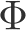The electrostatic potential, which generally will vary in space and different cellular compartments. See Ion Gradients.The concentration of species (or state) $\mathrm{X}$, generally equal to $N_X/V$. See States & Kinetics, Equilibrium.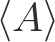Thermodynamic average of the quantity $A$ (under specified conditions). See Free Energy & Work.Helmholtz free energy, $\langle E \rangle - TS$ . See Free Energy & Work.Boltzmann's constant. $k_B \, T \sim R T$ is the natural scale of thermal energy at temperature $T$. See The Ideal Gas.Rate constant for transitions from $i$ to $j$. Probability per unit time to transition from $i$ to $j$. See Mass-Action Kinetics, States & Kinetics, Catalysis, Equilibrium.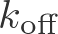Rate constant for unbinding - i.e., for bi-molecular dissociation. Probability per $s$ for a bi-molecular complex to dissociate. See Mass-Action Kinetics, Activated Carriers.Rate constant for binding - i.e., for bi-molecular association. Probability per $s$ for a single molecule to bind one molecule of another species, with the latter at the reference 1M concentration. See Mass-Action Kinetics, Activated Carriers.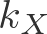Rate constant for a process "X", which might be a chemical reaction, isomerization, or (un)binding process - and should be determined by the context. See Mass-Action Kinetics, States & Kinetics, Catalysis, Synthesis.Number of systems or molecules in state $i$. See States & Kinetics, Equilibrium.Number of copies of species (e.g., molecule) $\mathrm{X}$. See States & Kinetics, Equilibrium.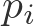Probability of state $i$, generally equal to $N_i/N$. See States & Kinetics, Equilibrium.The gas constant. $k_B \, T = R T$ is the natural scale of thermal energy at temperature $T$. See The Ideal Gas.The configuration of a system of $N$ atoms or molecules, it is short-hand for the full set of Cartesian coordinates: $\mathbf{r}^N = \{ x_1, y_1, z_1, \, x_2, y_2, z_2, \, \ldots, \, x_N, y_N, z_N \}$. See The Ideal Gas, Free Energy Storage in a Concentration Gradient.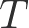Absolute temperature, in degrees Kelvin. See Free Energy & Work, The Ideal Gas.Volume of system. See The Ideal Gas, Free Energy Storage in a Concentration Gradient.

# Notation

## Notation and Technical Terminology

Symbol or TermDefinitionThe electrostatic potential, which generally will vary in space and different cellular compartments. See Ion Gradients.The concentration of species (or state) $\mathrm{X}$, generally equal to $N_X/V$. See States & Kinetics, Equilibrium.Thermodynamic average of the quantity $A$ (under specified conditions). See Free Energy & Work.Helmholtz free energy, $\langle E \rangle - TS$ . See Free Energy & Work.Boltzmann's constant. $k_B \, T \sim R T$ is the natural scale of thermal energy at temperature $T$. See The Ideal Gas.Rate constant for transitions from $i$ to $j$. Probability per unit time to transition from $i$ to $j$. See Mass-Action Kinetics, States & Kinetics, Catalysis, Equilibrium.Rate constant for unbinding - i.e., for bi-molecular dissociation. Probability per $s$ for a bi-molecular complex to dissociate. See Mass-Action Kinetics, Activated Carriers.Rate constant for binding - i.e., for bi-molecular association. Probability per $s$ for a single molecule to bind one molecule of another species, with the latter at the reference 1M concentration. See Mass-Action Kinetics, Activated Carriers.Rate constant for a process "X", which might be a chemical reaction, isomerization, or (un)binding process - and should be determined by the context. See Mass-Action Kinetics, States & Kinetics, Catalysis, Synthesis.Number of systems or molecules in state $i$. See States & Kinetics, Equilibrium.Number of copies of species (e.g., molecule) $\mathrm{X}$. See States & Kinetics, Equilibrium.Probability of state $i$, generally equal to $N_i/N$. See States & Kinetics, Equilibrium.The gas constant. $k_B \, T = R T$ is the natural scale of thermal energy at temperature $T$. See The Ideal Gas.The configuration of a system of $N$ atoms or molecules, it is short-hand for the full set of Cartesian coordinates: $\mathbf{r}^N = \{ x_1, y_1, z_1, \, x_2, y_2, z_2, \, \ldots, \, x_N, y_N, z_N \}$. See The Ideal Gas, Free Energy Storage in a Concentration Gradient.Absolute temperature, in degrees Kelvin. See Free Energy & Work, The Ideal Gas.Volume of system. See The Ideal Gas, Free Energy Storage in a Concentration Gradient.